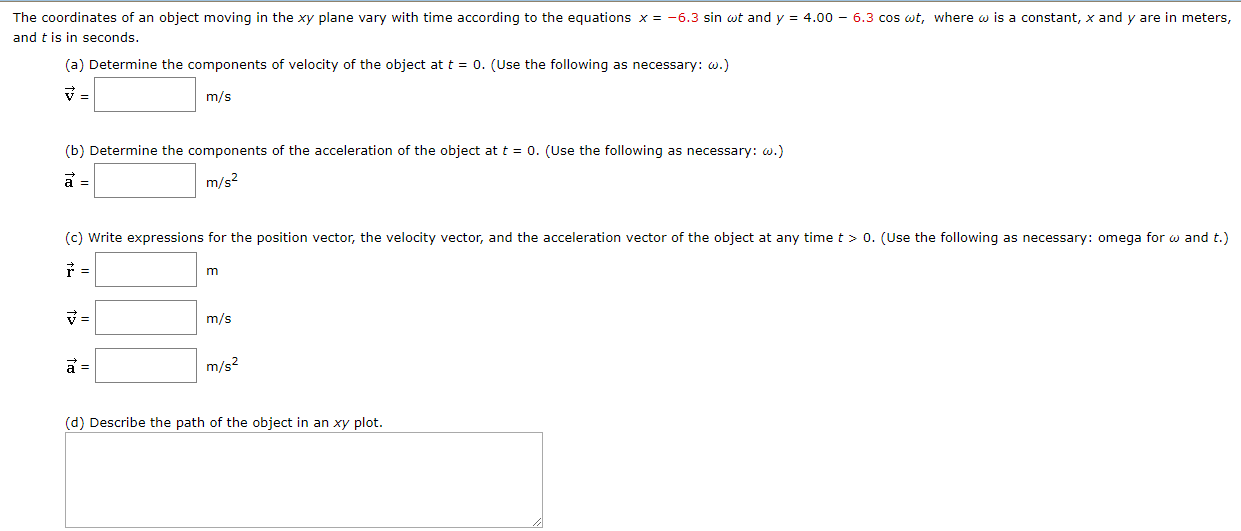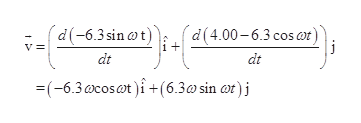# The coordinates of an object moving in the xy plane vary with time according to the equations x = -6.3 sin wt and yand t is in seconds4.00 - 6.3 cos wt, where w is a constant, x and y are in meters,(a) Determine the components of velocity of the object at t = 0. (Use the following as necessary: w.)m/s(b) Determine the components of the acceleration of the object at t0. (Use the following as necessary: w.)m/s2(c) Write expressions for the position vector, the velocity vector, and the acceleration vector of the object at any time t > 0. (Use the following as necessary: omega for w and t.)m/sm/s2(d) Describe the path of the object in an xy plot

Question
6 views

this is not the actual problem it is just the practice one, I just need a step by step on how to solve it because my teacher did not really teach this in class. thank you for any help you are able to provide.help_outlineImage TranscriptioncloseThe coordinates of an object moving in the xy plane vary with time according to the equations x = -6.3 sin wt and y and t is in seconds 4.00 - 6.3 cos wt, where w is a constant, x and y are in meters, (a) Determine the components of velocity of the object at t = 0. (Use the following as necessary: w.) m/s (b) Determine the components of the acceleration of the object at t 0. (Use the following as necessary: w.) m/s2 (c) Write expressions for the position vector, the velocity vector, and the acceleration vector of the object at any time t > 0. (Use the following as necessary: omega for w and t.) m/s m/s2 (d) Describe the path of the object in an xy plot fullscreen
check_circle

star
star
star
star
star
1 Rating
Step 1

(a)

the componet for velocity is

Step 2

substitute the expression for x and y in the above equation to calculate the velocity vectorhelp_outlineImage Transcriptionclose(d(-6.3sin ot) (d(4.00-6.3 cos ar) i V dt dt (-6.3 cosat +(6.3a sin at)j fullscreen
Step 3

substitute t=0 in the expressi...

### Want to see the full answer?

See Solution

#### Want to see this answer and more?

Solutions are written by subject experts who are available 24/7. Questions are typically answered within 1 hour.*

See Solution
*Response times may vary by subject and question.
Tagged in

### Vectors and Scalars